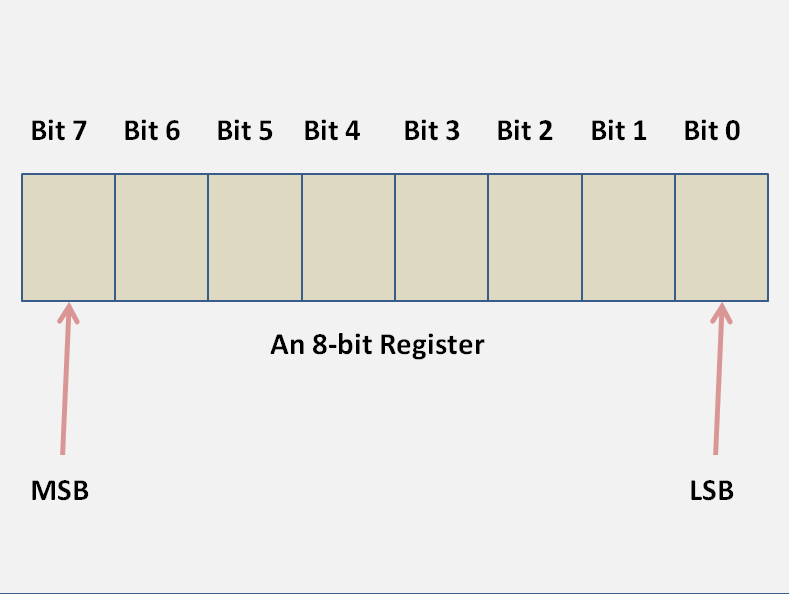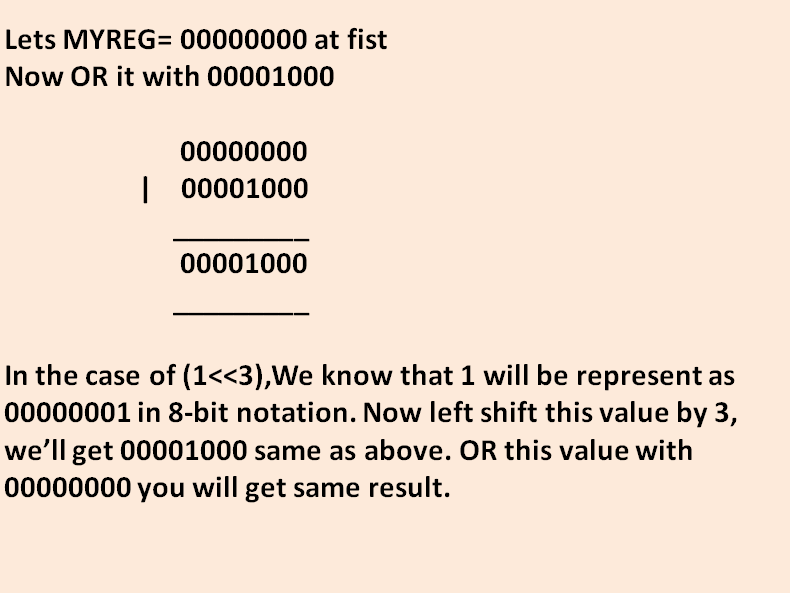# Embedded C : Programming in C for Embedded Systems

###### Embedded C, Programming in C, Embedded Systems, Hexadecimal in C, Binary value representation in C, bit-wise AND, bit-wise OR operation in C

Embedded C is not different or any subset of the original C. It’s just the programming way you use to develop the applications for the embedded systems. In Embedded C we use C to control the hardware by manipulating the microcontroller’s or flash memories or any other hardware’s registers. We write, we read and then based on the result we make our logic.

If you know how to program using C then you are ready for the embedded development. You just need to know how to read or write a register and before that what is a register. Further in this tutorial I am going to explain everything you need to know as a beginner.

What is a Register?

A register is basically a collection of bits; it might be a collection of 8, 16 or 32 bits (specifically for MCUs) or 10, 12 bits (ADC, DAC etc.). Each bit of a register might control a special case or in total that collection of bits might give us some information, whatever it’s designed for.When you are going to develop an application for your embedded system, you control the register of your MCU. In your embedded system your MCU is connected to all other of your peripherals like ADC, DAC, character LCD, Graphical LCD, GSM modem and list go on. So you control the peripherals by modifying (writing) some register of your MCU or you know the current state of your peripherals by reading some register of your MCU.

So we can say that:

A CPU writes a register to:

• Configure its own basic operation or state
•  Configure the peripheral
• Command the peripheral
• Transfer data to the peripheral

A CPU reads a register to:

• Know its current state
• Know the current state of peripheral
• Receive data from the peripheral

As I explained that a register is a collection of bits so to manipulate a register you have to change the values of bits. As you know in a bit can have two values (one at a time) 0 or 1. So a register will be having a binary value.

Represent Binary Numbers in C

In C, we normally assign a value to a variable using ‘=’ operator, e.g data=100. We are assigning a decimal value 100 to variable data. Now thinks a register as a variable, it’s not different.

For example you have an 8-bit register ‘MYREG’ and you want to assign a value 10010101.  In C, you will assign a value to this register as follows:

MYREG=0b10010101;

Did you notice, I used a ‘0b’ before the value ‘10010101’? In C, you have to use ‘0b’ before your binary value. If you don’t use ‘0b’ then it will be treated like a decimal value. Similarly you assign 16 and 32 bit values to register.

Represent HEX Numbers in C

Each hexadecimal digit represents four binary digits (bits), and the primary use of hexadecimal notation is a human-friendly representation of binary-coded values in computing and digital electronics. One hexadecimal digit represents a nibble, which is half of an octet or byte (8 bits). For example, byte values can range from 0 to 255 (decimal), but may be more conveniently represented as two hexadecimal digits in the range 00 to FF.

For example, binary value 10010101 can be represented as 95 (1001->9 and 0101->5).

So, MYREG =0x95;

Now you know how to assign a value to register. So I am going to explain how to SET (write logical 1) or CLEAR (write a logical 0) a bit.

SET a BIT in Register:

In embedded setting a bit is making that bit’s value to logical 1.  Note that you’re going to change a single bit, not all the bits.  To preserve the values of all other bits and change only single bit you have to use ‘|’ (OR) operator.

For example, you want to SET the 3rd bit of your register MYREG then you will write:

MYREG=MYREG |0b00001000; <- in binary notation

MYREG= MYREG |0x08;             <-in Hexadecimal notation

MYREG =MYREG | (1<<3);          <-using left-shift operator

Any of above code will change the 3rd bit’s value to logical 1 leaving all other bits unchanged. OR (|) operator doing a bit-wise OR operation on the register’s bits. Refer the below table for the OR operation.

 X Y O/P 0 0 0 1 0 1 0 1 1 1 1 1A Practical Approach:

Use of left-shift operator will be more practical. Lets a bit in your register has a name START. Its location on the register is 3.

Now you can define this as

#define START 3

Now change the register bit using left-shift register as

MYREG =MYREG | (1<<START);

This will change the bit-3 to value 1. This approach makes your code more readable and portable. For rest of the tutorial we’ll use this approach only.  Need to explain? Ok.

Portability of code:

Suppose you have defined START bit as 3. Later you need to change this bit location to another one. Then you have to make a small change only on bit definition i.e.  #define START 3 to #define START 4 in your header file. You don’t need to look and change this bit in your rest of the code that is not possible if you use another two methods.

MYREG = MYREG |0b00001000; does not give any idea what this bit is doing. On the other hand MYREG=MYREG | (1<<START) clearly tells you that you are staring an operation. Also typing 0b00001000 or 0x80 every time consume more time as well as you need to calculate the value.

CLEAR a BIT in Register:

Making a bit’s logical value 0 is known as clearing that bit in the register. You have to use & (AND operator).  This will clear the 3rd bit (will make its value 0) while leaving all other bits unchanged. Refer the below table for the AND operation.

 X Y O/P 0 0 0 1 0 0 0 1 0 1 1 1

For example, you want to CLEAR the 3rd bit then,

MYREG = MYREG & ~ (1<<3);

Below diagram explains how this is being accomplished.How to Know Current Status of a Bit?

To know if a particular bit is zero or one (0 or 1), you have to AND it with its AND MASK. AND mask will be the value where that particular bit is 1. For example if you want to know the current status of 3rd bit then AND mask will be 0b00001000 or 0x08 or (1<<3). If you AND this value with current value of the register then result will be non zero if the current value of bit is 1 else it will be zero.

For example

if (MYREG & (1<<3))

{

//Current status of bit is one (1)

}

Else

{

//current status of bit is zero (0)

}

Well, after this you know how to manipulate a register for development of firmware for your embedded system. This is the first part of this tutorial. You are going to get more on this topic soon. If you have any doubts please let me know. If you like this post please share and like its Facebook page.

## 3 Thoughts to “Embedded C : Programming in C for Embedded Systems”

1.Anonymous

Helped a lot. Thanks

2.integratedexpert

Really appreciate to your work thank you sharing fundamental information

3. […] Embedded C : Programming in C for Embedded Systems […]